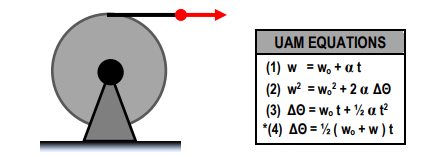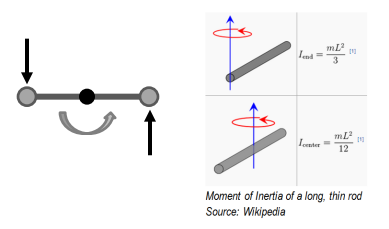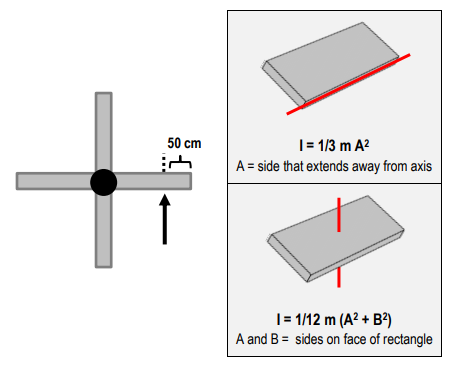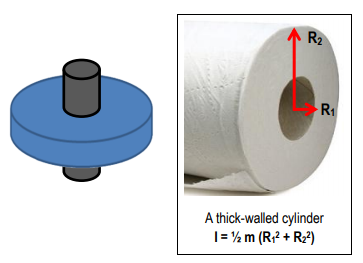Clutch Prep is now a part of Pearson
Ch 13: Rotational Inertia & EnergyWorksheetSee all chapters

# Torque with Kinematic Equations

See all sections
Sections
Parallel Axis Theorem
Intro to Rotational Kinetic Energy
Moment of Inertia of Systems
Conservation of Energy in Rolling Motion
Conservation of Energy with Rotation
Moment of Inertia via Integration
Energy of Rolling Motion
Moment of Inertia & Mass Distribution
Intro to Moment of Inertia
More Conservation of Energy Problems
Types of Motion & Energy
Torque with Kinematic Equations
Rotational Dynamics with Two Motions
Rotational Dynamics of Rolling Motion

Concept #1: Torque with Kinematic Equations

Transcript

Practice: A light, long rope is wrapped around a solid disc, in such a way that pulling the rope causes the disc to spin about a fixed axis perpendicular to itself and through its center. The disc has mass 40 kg, radius 2 m, and is initially at rest, and the rope unwinds without slipping. You pull on the rope with a constant 200 N. Use the rotational version of Newton’s Second Law to calculate how fast (in rad/s) the disc be spinning after you pull 50 m of rope.Practice: A system is made of two small, 3 kg masses attached to the ends of a 5 kg, 4 m long, thin rod, as shown. The system is free to rotate about an axis perpendicular to the rod and through its center. Two forces, both of magnitude F and perpendicular to the rod, are applied as shown below. What must the value of F be to the system from rest to 10 rad/s in exactly 8 complete revolutions?Practice: Two rotating doors, each 6.0 m long, are fixed to the same central axis of rotation, as shown (top view). When you push on one door with a constant 100 N, directed perpendicular from the face of the door and 50 cm from its outer edge, the rotating door system takes 8 s to complete a full revolution from rest. The doors can be modeled as thin rectangles (moments of inertia for thin rectangles, around two different axes, are shown for reference). Calculate the mass of the system.Example #1: Stopping flywheel with friction

Transcript